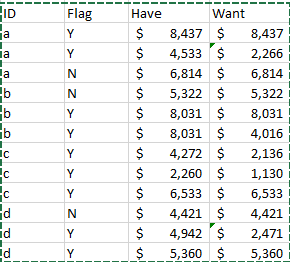## Keeping Max Value and Changing Other Values in Subgroup Contingent Upon Logic

In words here are the rules I want to implement:

If Flag = N then WANT = HAVE

If Flag = Y and HAVE value is max among observations with same ID, then WANT=HAVE

If Flag = Y and above rule is false, then WANT=.5*HAVE

If there's an instance where two values for an ID are max (and Flag=Y), then arbitrarily WANT = HAVE for one and WANT = .5*HAVE for the other. (this is shown for ID b below)1 ACCEPTED SOLUTION

Accepted Solutions

## Re: Keeping Max Value and Changing Other Values in Subgroup Contingent Upon Logic

``````proc sort data=have;
by ID flag have;
run;

data want;
set have;
by ID flag have;
if Flag = 'N' then want=have;
else if flag ='Y' and last.flag then want=have;
else want =.5*have;
run;``````
6 REPLIES 6

## Re: Keeping Max Value and Changing Other Values in Subgroup Contingent Upon Logic

``````proc sort data=have;
by ID flag have;
run;

data want;
set have;
by ID flag have;
if Flag = 'N' then want=have;
else if flag ='Y' and last.flag then want=have;
else want =.5*have;
run;``````

## Re: Keeping Max Value and Changing Other Values in Subgroup Contingent Upon Logic

This worked superbly. Thanks.

## Re: Keeping Max Value and Changing Other Values in Subgroup Contingent Upon Logic

Then kindly mark the question as answered.Ksharp
Super User

## Re: Keeping Max Value and Changing Other Values in Subgroup Contingent Upon Logic

You need clarify something more .

What If there's an instance where THREE values for an ID are max (and Flag=Y), What you are going to do ?

And Don't post it as picture, post it as TEXT . No one would like to type it for you and would ignore your quesiton.

``````data have;
input id \$ flag \$ have ;
cards;
a y 8437
a y 4533
a n 6814
b n 5322
b y 8031
b y 8031
;
run;
data want;
do until(last.id);
set have;
by id;
max=max(max,have);
end;
do until(last.id);
set have;
by id;
if flag='n' then want=have;
if flag='y' and have ne max then want=.5*have;
if flag='y' and have=max then do;
if found then want=.5*have;
else do;want=have;found=1;end;
end;
output;
end;
drop max;
run;``````

## Re: Keeping Max Value and Changing Other Values in Subgroup Contingent Upon Logic

@Ksharp, thanks for the feedback and the tip about text vs image.

It looks to me like @mohamed_zaki's reply addresses the issue of if three values are max and flag = 'Y'. The data would be sorted so that the HAVE column ascends so that

else if flag ='Y' and last.flag then want=have;
else want =.5*have;

causes only the last instance to set want=have, whereas the previous instances would be set to want=.5*have.

Am I incorrect?Ksharp
Super User

## Re: Keeping Max Value and Changing Other Values in Subgroup Contingent Upon Logic

It is hard to say something , it is all depend on what you want.

And There looks like some problem in his code :

data have;
input id \$ flag \$ have ;
cards;
a y 8437
a y 4533
a n 6814
b n 9322
b y 8031
b y 8031
;
proc sort data=have;
by ID flag have;
run;

data want;
set have;
by ID flag have;
if Flag = 'n' then want=have;
else if flag ='y' and last.flag then want=have;
else want =.5*have;
run;

Discussion stats
• 6 replies
• 1100 views
• 3 likes
• 3 in conversation